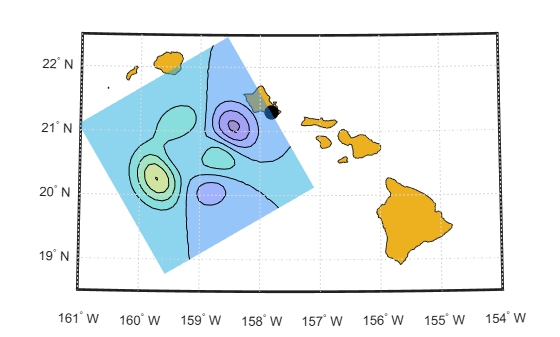# geocontourxy

Contour grid in local system with latitude-longitude results

## Syntax

``````[contourLines,contourPolygons] = geocontourxy(X,Y,Z,lat0,lon0,h0)``````
``[___] = geocontourxy(___,Name,Value)``

## Description

example

``````[contourLines,contourPolygons] = geocontourxy(X,Y,Z,lat0,lon0,h0)``` returns line and polygon geoshapes containing contour lines and contour fill polygons, respectively. This function is non-graphical. You can plot the return values using `geoshow`, if desired.```
````[___] = geocontourxy(___,Name,Value)` specifies name-value pairs that control aspects of the operation. Parameter names can be abbreviated and are case-insensitive.```

## Examples

collapse all

Define a set of X and Y coordinates and create contour lines and contour polygons.

```X = -150000:10000:150000; Y = 0:10000:300000; [xmesh, ymesh] = meshgrid(X/50000, (Y - 150000)/50000); Z = 8 + peaks(xmesh, ymesh); lat0 = dm2degrees([ 21 18]); lon0 = dm2degrees([-157 49]); h0 = 300; levels = 0:2:18; [contourLines, contourPolygons] = geocontourxy(X,Y,Z,lat0,lon0,h0, ... 'LevelList',levels,'XYRotation',120)```
```contourLines = 8x1 geoshape vector with properties: Collection properties: Geometry: 'line' Metadata: [1x1 struct] Vertex properties: (8 features concatenated with 7 delimiters) Latitude: [21.1171 21.0296 20.9819 20.9814 20.9880 21.0702 21.1122 ... ] Longitude: [-158.5364 -158.5053 -158.4015 -158.3987 -158.3903 ... ] Height: [769.9110 771.5545 686.9961 684.5002 672.2181 584.6248 ... ] Feature properties: ContourLevel: [2 4 6 8 10 12 14 16] ```
```contourPolygons = 9x1 geoshape vector with properties: Collection properties: Geometry: 'polygon' Metadata: [1x1 struct] Vertex properties: (9 features concatenated with 8 delimiters) Latitude: [21.1171 21.1630 21.1671 21.1431 21.1122 21.0702 20.9880 ... ] Longitude: [-158.5364 -158.5114 -158.4450 -158.3876 -158.3542 ... ] Height: [769.9110 725.7670 650.4579 599.1402 578.2085 584.6248 ... ] Feature properties: LowerContourLevel: [0 2 4 6 8 10 12 14 16] UpperContourLevel: [2 4 6 8 10 12 14 16 18] ```

Display Hawaii on a map, add a marker, and then display the polygons returned by `geocontourxy` on the map.

```figure usamap([18.5 22.5],[-161 -154]) hawaii = shaperead('usastatehi.shp', 'UseGeoCoords', true,... 'Selector',{@(name) strcmpi(name,'Hawaii'), 'Name'}); geoshow(hawaii) geoshow(lat0,lon0,'DisplayType','point','Marker','o',... 'MarkerEdgeColor','k','MarkerFaceColor','k','MarkerSize',10) cmap = parula(1 + length(levels)); for k = 1:length(contourPolygons) lat = contourPolygons(k).Latitude; lon = contourPolygons(k).Longitude; geoshow(lat,lon,'Display','polygon', ... 'FaceColor',cmap(k,:),'FaceAlpha',0.5,'EdgeColor','none') end geoshow(contourLines.Latitude,contourLines.Longitude,'Color','black')```## Input Arguments

collapse all

X-component of a mesh that locates each element of Z in a local x-y plane, specified as a vector or matrix. `geocontourxy` assumes that units are meters unless you provide a `Spheroid` input, in which case the units of your input must match the`LengthUnit` property of the `Spheroid` object.

Data Types: `single` | `double`

Y-component of a mesh that locates each element of Z in a local x-y plane. specified as a vector or matrix. `geocontourxy` assumes that units are meters unless you provide a `Spheroid` input, in which case the units of your input must match the`LengthUnit` property of the `Spheroid` object.

Data Types: `single` | `double`

Data to be contoured, specified as a 2-D array.

Data Types: `single` | `double` | `int8` | `int16` | `int32` | `int64` | `uint8` | `uint16` | `uint32` | `uint64`

Geodetic latitude of local origin (reference) point, specified as a scalar value in units of degrees.

Data Types: `single` | `double`

Geodetic longitude of local origin (reference) point, specified as a scalar value in units of degrees.

Data Types: `single` | `double`

Ellipsoidal height of local origin (reference) point, specified as a scalar value. `geocontourxy` assumes that units are meters unless you provide a `Spheroid` input, in which case the units of your input must match the unit specified in the`LengthUnit` property of the `Spheroid` object.

Data Types: `single` | `double`

### Name-Value Arguments

Specify optional comma-separated pairs of `Name,Value` arguments. `Name` is the argument name and `Value` is the corresponding value. `Name` must appear inside quotes. You can specify several name and value pair arguments in any order as `Name1,Value1,...,NameN,ValueN`.

Example: `[contourLines, contourPolygons] = geocontourxy(X,Y,Z,lat0,lon0,h0,'LevelList',levels,'XYRotation',120)`

Contour levels, specified as a vector of Z-values. By default, the `geocontourxy` function chooses levels that span the range of values.

Data Types: `single` | `double` | `int8` | `int16` | `int32` | `int64` | `uint8` | `uint16` | `uint32` | `uint64`

Rotation angle of the local x-y system, measured counterclockwise from the xEast-yNorth system, specified as a scalar value in units of degrees.

Data Types: `single` | `double`

Reference spheroid, specified as a `referenceEllipsoid`, `oblateSpheroid`, or `referenceSphere` object. Use the constructor for one of these three classes, or the `wgs84Ellipsoid` function, to construct a Mapping Toolbox spheroid object. (You cannot directly pass in to `geocontourxy` the name of your spheroid. Instead, pass that name to `referenceEllipsoid` or `referenceSphere` and use the resulting object.) By default, `geocontourxy` uses the WGS84 reference ellipsoid with units of meters.

## Output Arguments

collapse all

Contour lines, returned as a line `geoshape` with one element per contour level. `Latitude` and `Longitude` properties contain contour line vertices in degrees. The contour level value of the k-th element is stored in the `ContourLevel` feature property of `contourLines(k)`. A third vertex property, `Height`, contains the ellipsoidal height of each vertex. In combination with `Latitude` and `Longitude`, it completes the definition of the 3-D location of the contour line in the plane that contains the local origin and is parallel to the tangent plane at the origin latitude and longitude.

Contour polygons, returned as a polygon geoshape with one element (contour fill polygon) per contour interval. `Latitude` and `Longitude` properties contain the vertices of the contour fill polygons, specified in degrees. The `LowerContourLevel` and `UpperContourLevel` properties of `contourPolygons(k)` store the limits of the k-th contour interval. As in the case of lines, a third vertex property, `Height`, is included.

### Topics

Introduced in R2016a

## SupportGet trial now